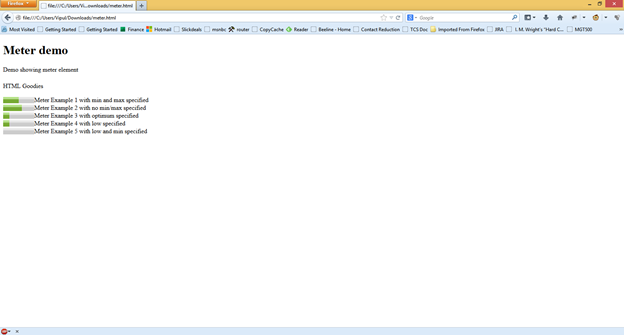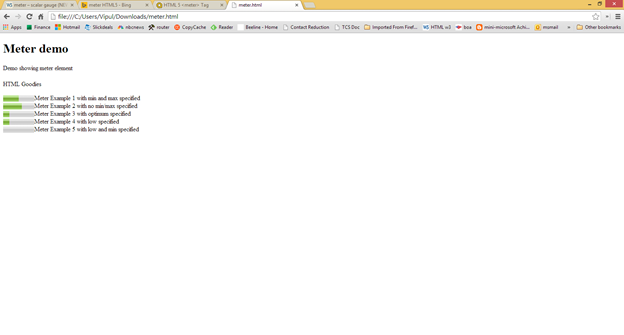# Basics About the New HTML5 Meter Markup Element

### WEBINAR:On-Demand

Desktop-as-a-Service Designed for Any Cloud ? Nutanix Frame

# Introduction

The meter markup element is a new markup introduced as part of the HTML5 specification which is intended to be used to represent a measurement to the users.

# HTML5 specification says …

The HTML5 specifications describes the meter markup element as an element which represents a scalar gauge providing a measurement within a known range, or fractional value.

Source: http://www.w3.org/TR/html-markup/meter.html#meter

The DOM interface for the meter markup element is

`interface HTMLMeterElement : HTMLElement {`
`      attribute double value;`
`      attribute double min;`
`      attribute double max;`
`      attribute double low;`
`      attribute double high;`
`      attribute double optimum;`
` readonly attribute NodeList labels;`
`};`

The meter element is intended to be used where we want to represent a fixed value which is part of a range. This is unlike the progress markup element (http://www.htmlgoodies.com/html5/markup/showing-progress-in-your-html5-pages-with-the-progress-markup-element.html#fbid=27uh4K9fMvd) which is used to represent a value which is changing.

The meter markup element supports phrasing content.

Besides the global attributes, the meter markup element supports the following specific attributes:

• value - represents the "measured" value by the meter. It can accept any floating-point number.
• min - represents the lower bound range for the meter. It can accept any floating-point number.
• low - represents the point that marks the upper boundary of the "low" segment of the meter. It can accept any floating-point number.
• high - represents the point that marks the lower boundary of the "high" segment of the meter. It can accept any floating-point number.
• max - represents the upper bound of the range for the meter. It can accept any floating-point number.
• optimum - represents the point that marks the "optimum" position for the meter.

There are certain constraints with how the meter markup element can be used.

• A meter markup element cannot contain another meter markup element.
• "value" is required attribute.
• "min" <= "value" when "min" is declared.
• "value" >= "0" when min is absent
• "value" <= "max" when "max" is declared.
• "value" <= "1" when "max" is absent
• "min" <= "max" when both are declared.
• "max" >= "0" when "min" is absent.
• "min" <= "1" when "max" is absent.
• "min" <= "low" when both are declared.
• "low" >= "0" when "min" is absent.
• "min" <= "high" when both are declared.
• "high" >= "0" when "min" is absent.
• "low" <= "high" when both are declared.
• "high" <= "max" when both are declared.
• "high" <= "1" when "max" attribute is absent.
• "low" <= "max" when both are declared.
• "low" <= "1" when "max" attribute is absent.
• "min" <= "optimum" when both are declared.
• "optimum" >= "0" when "min" attribute is absent.
• "optimum" <= "max" when both are declared.
• "optimum" <= "1" when "max" attribute is absent

As we can see from the above list, there are a number of constraints for using the meter elements.

# Hands On

Let us create a simple HTML5 web page which uses the meter markup element.

// Meter.html

<!DOCTYPE html>

<html>

<body>

<h1>Meter demo</h1>

<p>Demo showing meter element</p>

<footer>

<h1></h1>

<p>HTML Goodies</p>

</footer>

<meter value="5" min="0" max="10">Meter markup with min and max specified</meter>Meter Example 1 with min and max specified<br>

<meter value="0.6">Meter markup with no min/max specified</meter>Meter Example 2 with no min/max specified<br>

<meter value="2" min="0" max="10" optimum="6">Meter markup with optimum specified</meter>Meter Example 3 with optimum specified<br>

<meter value="2" low="0" max="10">Meter markup with optimum specified</meter>Meter Example 4 with low specified<br>

<meter value="2" min="0" low="1" max="10">Meter markup with optimum specified</meter>Meter Example 5 with low and min specified<br>

</body>

</html>?

In the above HTML snippet, we have create a simple HTML5 web page which demonstrates use of meter markup element.

Internet Explorer 11 does not render this. But FireFox and Google Chrome render the meter markup element.

In Firefox,# Where to use meter markup element

The meter markup element can be used in the following scenarios:

1. To demonstrate survey results (when the responses are graded on a scale)
2. To display remaining capacity of a hard drive, user account, spending limit, etc.

# Summary

In this article, we learned the basics about the meter markup element. I hope you have found this information useful.

# About the author

Vipul Patel is a Program Manager currently working at Amazon Corporation. He has formerly worked at Microsoft in the Lync team and in the .NET team (in the Base Class libraries and the Debugging and Profiling team). He can be reached at vipul.patel@hotmail.com

• #### Web Development Newsletter Signup

Invalid email
You have successfuly registered to our newsletter.
•
•
•Thanks for your registration, follow us on our social networks to keep up-to-date